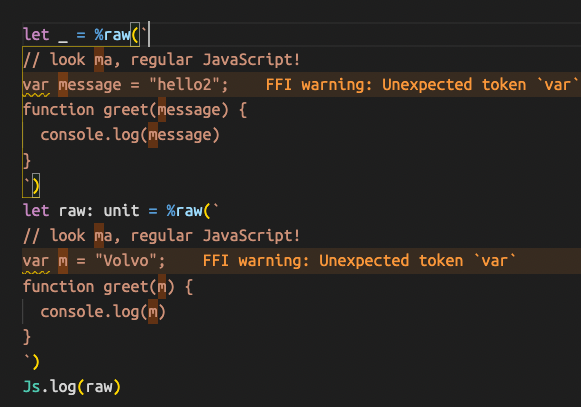The following example from the docs get an error stating `the arguments a, b will not be used in the function. Also, the logging output is `[function anonymout]`

``````
function(a, b) {
console.log("hello from raw JavaScript!");
return a + b
}
`)

``````

Changing it to any of these yields the same result:

``````let add: (int, int) => unit = (a: int, b: int) =>
%raw(`
function(a, b) {
return a + b
}
`)

let add: (int, int)=>unit => int = (a: int, b: int) =>
%raw(`
function(a, b) {
return a + b
}
`)

let x: unit =>int = add(1, 2)
Js.log(x)

[Function (anonymous)]
[Function (anonymous)]
[Function (anonymous)]
``````

What is the proper syntax for creating and calling this function?

Thanks

It works fine in the playground? You might have some stale compiler version. From the sound of it, you’ve also been accidentally currying some stuff.

Btw, we highly recommend you to keep your JS output tab open so that you can see what you’ve written.

Maybe you meant this? Your latter examples kinda do more than your first one

``````let add: (int, int) => unit =
%raw(`
function(a, b) {
return a + b
}
`)
``````
1 Like

@hoichi thanks. that works.

``````let add: (int, int) => unit =
%raw(`
function(a, b) {
return a + b
}
`)
``````

Seems like docs need to be updated.

Not sure what is going on with the other raw example. Im getting an error on the Javascript keyword raw when copying the example to an editor.`%%raw` lets you embed top-level raw JS code, `%raw` lets you embed expression-level

The code in the screen shot is not an expression I guess.

1 Like

The docs are up to date. As I said, please read them and check the first link you posted, provide the compiler version, and keep the output tab open.

Regarding your error: you’ve used `%raw` which is not a top-level extension point: Attribute (Decorator) | ReScript Language Manual. Check the output and you’ll see. It doesn’t make sense to have a `var` statement inside a `let` statement.

Thanks for the extension point link. Not something I paid attention to.

For whatever reason I thought the example code,

``````%%raw(`
// look ma, regular JavaScript!
var message = "hello";
function greet(m) {
console.log(m)
}
`)
``````

was supposed to run given there is a link to the playground. Maybe that just to show it compiles. You have to bind to it to to access it then.

``````%%raw(`
// look ma, regular JavaScript!
var message = "hello";
function greet(m) {
console.log(m)
}
`)
@val
external greet: (string) => unit = "greet"
let _ = greet("me")

@val
external message: string = "message"
Js.log(message)
``````

The link at the bottom of the page for future reference.